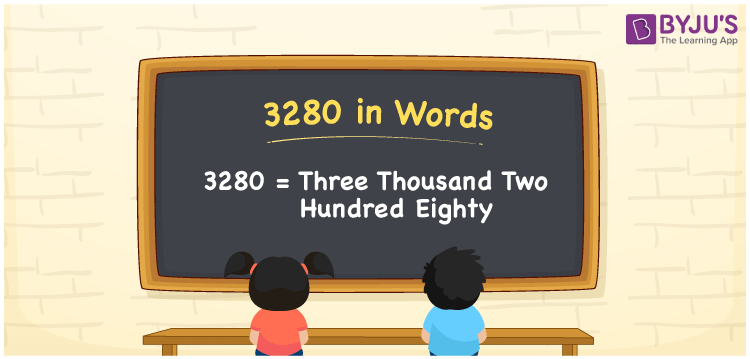# 3280 in Words

3280 in words is written as Three thousand two hundred eighty. In both the International System of Numerals and the Indian System of Numerals, 3280 is written as Three thousand two hundred eighty. The number 3280 is a Cardinal Number as it represents some quantity. For example, “that flower vase costs 3280 rupees”.

 3280 in Words Three thousand two hundred eighty Three thousand two hundred eighty in Number 3280

## 3280 in English Words

3280 in English words is read as “Three thousand two hundred eighty”.## How to Write 3280 in Words?

To write 3280 in words, we shall use the place value chart. In the place value chart, put 3 in the thousands, 2 in the hundreds, 8 in the tens, and 0 in the ones, respectively. Let us make a place value chart to write the number 3280 in words.

 Thousands Hundreds Tens Ones 3 2 8 0

Thus, we can write the expanded form as

3 × Thousand + 2 × Hundred + 8 × Ten + 0 × One

= 3 × 1000 + 2 × 100 + 8 × 10 + 0 × 1

= 3000 + 200 + 80 + 0

= 3280

= Three thousand two hundred eighty.

3280 is a natural number that is the successor of 3279 and the predecessor of 3281.

3280 in words – Three thousand two hundred eighty

• Is 3280 an odd number? – No
• Is 3280 an even number? – Yes
• Is 3280 a perfect square number? – No
• Is 3280 a perfect cube number? – No
• Is 3280 a prime number? – No
• Is 3280 a composite number? – Yes

## Frequently Asked Questions on 3280 in Words

Q1

### How to write 3280 in words?

3280 in words is written as Three thousand two hundred eighty.
Q2

### How to write 3280 in the International and Indian System of Numerals?

In both, the system of numerals, 3280 in words, is written as Three thousand two hundred eighty.
Q3

### How to write 3280 in a place value chart?

In the place value chart, write 3 in the thousands, 2 in the hundreds, 8 in the tens, and 0 in the ones, respectively.